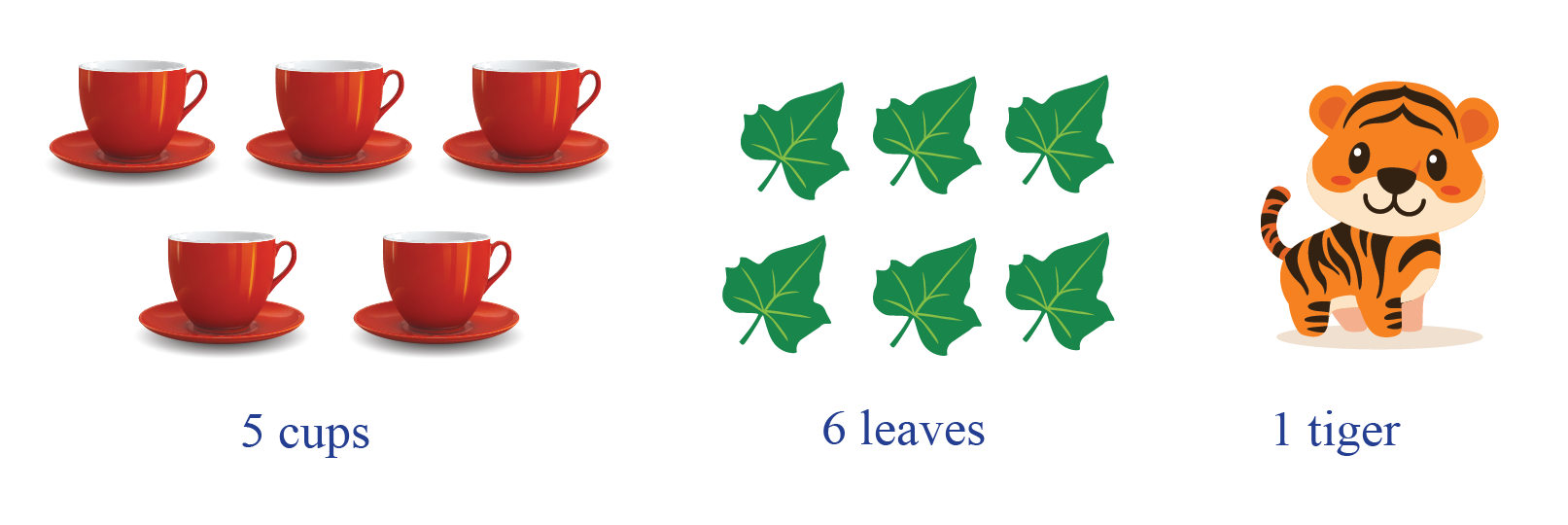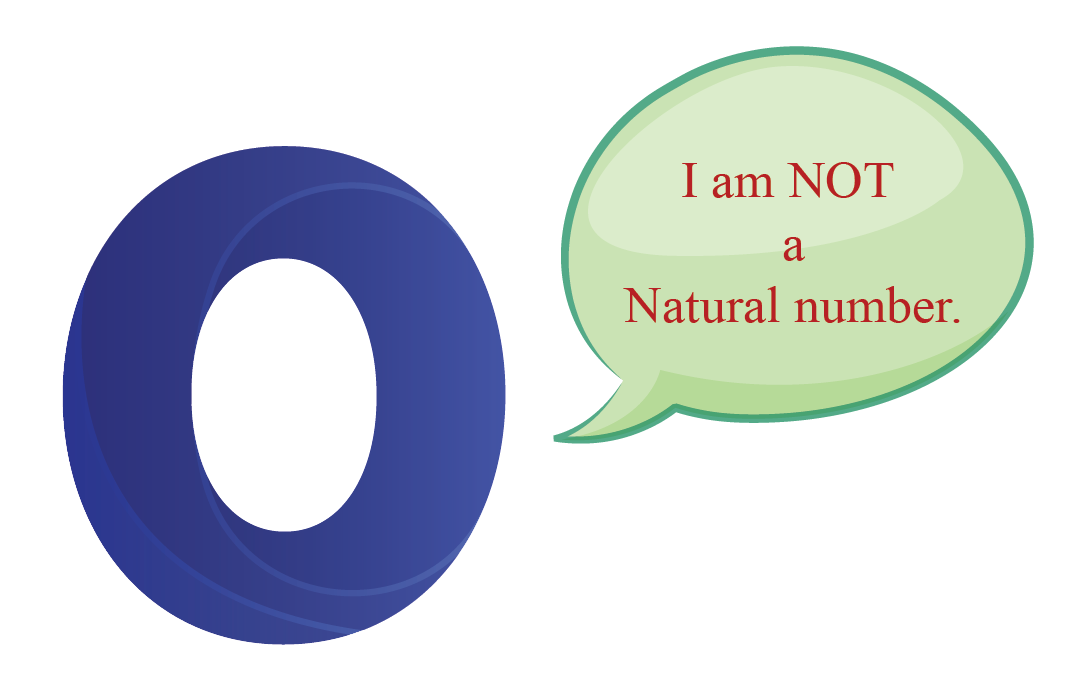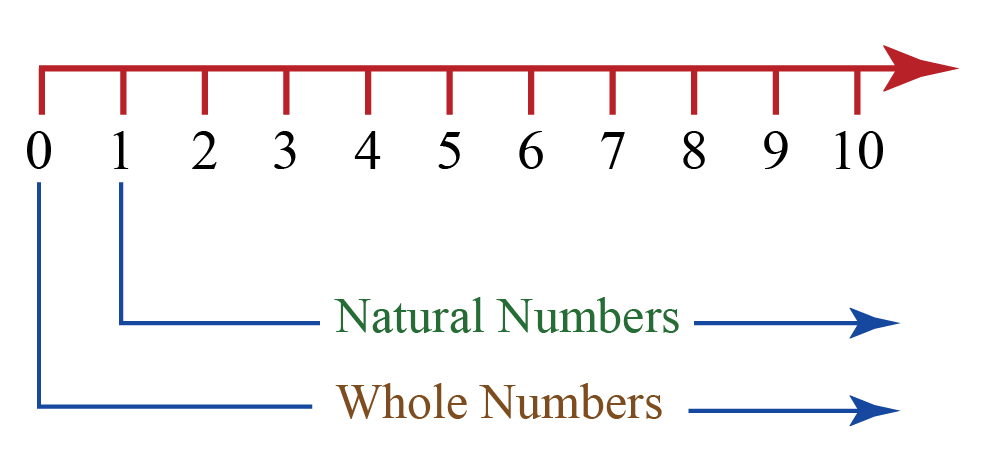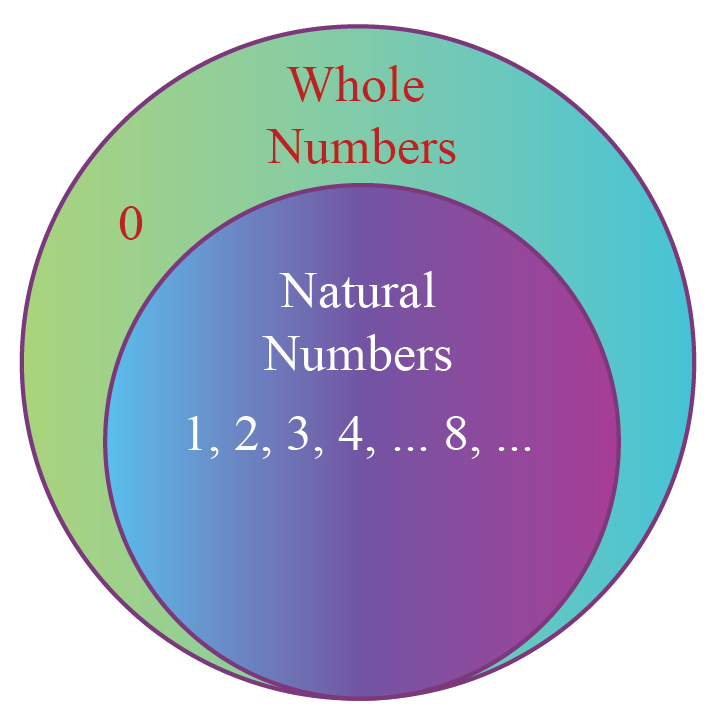# Natural Numbers

Natural Numbers
Go back to  'Numbers'

 1 Concept Video on Natural Numbers 2 Introduction to Natural Numbers 3 Natural Numbers 4 Whole Numbers 5 Natural Numbers and Whole Numbers 6 Properties of Natural Numbers 7 Thinking out of the Box! 8 Solved Examples on Natural Numbers 9 Important Notes on Natural Numbers 10 Practice Questions on Natural Numbers 11 Important Topics of Natural Numbers 12 Maths Olympiad Sample Papers 13 Frequently Asked Questions (FAQs) 14 FREE Worksheets of Natural Numbers

We at Cuemath believe that Math is a life skill. Our Math Experts focus on the “Why” behind the “What.” Students can explore from a huge range of interactive worksheets, visuals, simulations, practice tests, and more to understand a concept in depth.

Book a FREE trial class today! and experience Cuemath’s LIVE Online Class with your child.

## Understanding Natural Numbers

Before we begin explaining Natural Numbers, let us first get an idea of the concept with the help of this simple video:

## Introduction

We see numbers everywhere around the world.

• For counting the objects, we use numbers.
• For representing money, we use numbers.
• For measuring the temperature, we use numbers, etc.

Especially, the numbers that are used for counting objects are called “natural numbers”.

While counting objects, we say like $$5$$ cups, $$6$$ leaves, $$1$$ tiger, etc.## Natural Numbers

### Definition of Natural Numbers

Natural numbers are the numbers that are used for counting.

The set of natural numbers in Mathematics is the set $$\textbf{ \{1,2,3,...\} }$$.

Recall that a set is a collection of elements (numbers in this context).

The set of natural numbers is denoted by the symbol, $$N$$.

 N = $$\{1,2,3,4,5,...\}$$

### Smallest Natural Number

We note that the smallest element in $$N$$ is 1 and that for every element in $$N$$, we can talk about the next element in terms of 1 and $$N$$ (which is 1 more than that element).

For example, two is one more than one, three is one more than two and so on.

### Natural Numbers from 1 to 100

The natural numbers from $$1$$ to $$100$$ are 1, 2, 3, 4, 5, 6, 7, 8, 9, 10, 11, 12, 13, 14, 15, 16, 17, 18, 19, 20, 21, 22, 23, 24, 25,26, 27, 28, 29, 30, 31, 32, 33, 34, 35, 36, 37, 38, 39, 40, 41, 42, 43, 44, 45, 46, 47, 48, 49, 50, 51, 52, 53, 54, 55, 56, 57, 58, 59, 60, 61, 62, 63, 64, 65, 66, 67, 68, 69, 70, 71, 72, 73, 74, 75, 76, 77, 78, 79, 80, 81, 82, 83, 84, 85, 86, 87, 88, 89, 90, 91, 92, 93, 94, 95, 96, 97, 98, 99 and 100

Enter a number in the box below and you will see those many natural numbers.

### Is 0 a Natural Number?

No, $$0$$ is NOT a natural number because natural numbers are counting numbers. For counting any number of objects, we start counting from $$1$$ and not from $$0$$.### Odd Natural Numbers

The odd natural numbers are the numbers which are odd and belong to the set $$N$$. So the set of odd natural numbers is $$\{1,3,5,7,...\}$$.

### Even Natural Numbers

The even natural numbers are the numbers which are even and belong to the set $$N$$. So the set of even natural numbers is $$\{2,4,6,8,...\}$$.

## Whole Numbers

The set of whole numbers is as same as the set of natural numbers, except it includes an additional number which is $$0$$.

The set of whole numbers in Mathematics is the set $$\textbf{ \{0,1,2,3,...\} }$$. It is denoted by the letter, $$W$$

 W = $$\{0,1,2,3,4…\}$$

## Natural Numbers and Whole Numbers

From the above definitions, we can understand that every natural number is a whole number.

Also, every whole number other than $$0$$ is a natural number.

The set of natural numbers and the set of whole numbers can be shown on the number line as follows:We can say that the set of natural numbers is a subset of the set of whole numbers.### Comparison between Natural numbers and Whole numbers

Natural Number Whole Number
The set of natural numbers is, $$N= \{1,2,3,...\}$$ The set of whole numbers is, $$W=\{0,1,2,3,...\}$$
The smallest natural number is $$1$$ The smallest whole number is $$0$$
We cannot determine the largest natural number We cannot determine the largest whole number
Each natural number is obtained by adding $$1$$ to its previous number Each whole number is obtained by adding $$1$$ to its previous number
$$0$$ is NOT a natural number $$0$$ is a whole number

## Properties of Natural Numbers

### 1. Closure Property:

The sum and product of two natural numbers is always a natural number.

The closure property of $$N$$ is stated as follows:

For all $$a,b \in N$$

 $a + b \in N\\ {\text{ and }}\\a \times b \in N$

So the set of natural numbers, $$N$$ is closed under addition and multiplication.

### 2. Associative Property:

The sum or product of any three natural numbers remains the same though the grouping of numbers is changed.

The associative property of $$N$$ is stated as follows:

For all $$a,b,c \in N$$

 $\begin{gathered} a + \left( {b + c} \right) = \left( {a + b} \right) + c \\ {\text{and}} \\ a \times \left( {b \times c} \right) = \left( {a \times b} \right) \times c \\ \end{gathered}$

So the set of natural numbers, $$N$$ is associative under addition and multiplication.

### 3. Commutative Property:

The sum or product of two natural numbers remains the same even after interchanging the order of the numbers.

The commutative property of $$N$$ is stated as follows:

For all $$a,b \in N$$

 $\begin{gathered} a + b = b + a \\ {\text{and}} \\ a \times b = b \times a \\ \end{gathered}$

So the set of natural numbers, $$N$$ is commutative under addition and multiplication.

Let us summarise these three properties of natural numbers in a table.

Operation Closure Property Associative Property Commutative Property
Subtraction no no no
Multiplication yes yes yes
Division no no no

### 4. Distributive Property:

The distributive property of multiplication over addition is

 \begin{align}a \times (b+c) &= a \times b + a \times c\end{align}

The distributive property of multiplication over subtraction is

 \begin{align}a \times (b-c) = a \times b - a \times c \end{align}Think Tank
1. Is $$N$$ closed under subtraction and division?
2. Is $$N$$ associative under subtraction and division?
3. Is $$N$$ commutative under subtraction and division?

Help your child score higher with Cuemath’s proprietary FREE Diagnostic Test. Get access to detailed reports, customised learning plans and a FREE counselling session. Attempt the test now.

## Solved Examples

 Example 1

Identify the natural numbers among the following numbers.

$$-1, 0, 3, \dfrac{1}{2}, 5$$.

Solution:

The set of natural numbers in Mathematics is the set $$\textbf{1, 2, 3, ...}$$

So among the given numbers, the natural numbers are, $$3 \text{ and }5$$.

 Example 2

Identify the natural numbers among the following numbers.
$$7, 2, -3, -\dfrac{3}{5}, 0$$.

Solution:

The set of natural numbers in Mathematics is the set $$\textbf{1, 2, 3, ...}$$

So among the given numbers, the whole numbers are, $$7, \text{ and }2$$.

Numbers and Number Systems
Numbers and Number Systems
grade 9 | Questions Set 2
Numbers and Number Systems